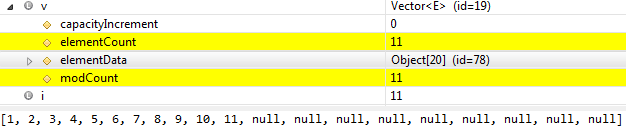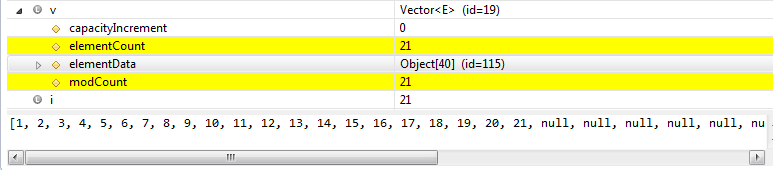### what is the default initial capacity of VECTOR, how it is resized and size is increased in java

In this Collection framework tutorial we will learn what is the default initial capacity of VECTOR, how it is resized and size is increased in java.

Contents of page >
• 1) What is meaning of capacity in Vector in java?
• 2) Does size of Vector grows automatically in java?
• 3) What is default initial capacity of Vector in java?
• 4) By what size Vector is resized in java? How much size increases when Vector is resized in java?
• 5) But how Vector is resized in java?
• 6) Let’s see java program to see what is default initial capacity of Vector in java and how it is resized in java by putting java application debug mode?
• 7) Can we change default initial capacity of Vector in java?
• 8) Can we change resizing of Vector in java? The amount by which the capacity of Vector is increased when the Vector overflows?
• 9) Should you change default initial capacity of Vector in java?
• 10) How Vector is implemented in java?

1) What is meaning of capacity in Vector in java?
Capacity is the size of the array which is used to store elements in the Vector.

2) Does size of Vector grows automatically in java?
Yes, size of Vector grows automatically in java. Vector resizes itself dynamically in java.

3) What is default initial capacity of Vector in java?
Default initial capacity of Vector is 10.

java.util.Vector default constructor defines size of 10.
 /**     * Constructs an empty vector so that its internal data array has size 10     */    public Vector() {        this(10);    }

4) By what size Vector is resized in java? How much size increases when Vector is resized in java?
Vector is resized by 100% of it’s current size.
So, Vector will be resized from 10, to 20, to 40, to 80 and so on.

5) But how Vector is resized in java?
Vector’s add method internally calls ensureCapacityHelper method, which calls grow method, grow method >
• creates new array of higher capacity and
• copies existing array to new one and
• return the new array.

6) Let’s see java program to see what is default initial capacity of Vector in java and how it is resized in java by putting java application debug mode?
 import java.util.Vector; import java.util.List; public class VectorDefaultCapacityAndResizing { public static void main(String args[]) {      List Vector = new Vector();      for (int i = 1; i < 25; i++) {           Vector.add(i);      } } }

When, new Vector<Integer>() is executed, Size of Vector is 10.Till addition of 10th element size of Vector remains same.

As soon as 11th element is added, using add(i), where i=11, Vector is resized to 20.Till addition of 20th element size of Vector remains same.

As soon as 21th element is added, using add(i), where i=21, Vector is resized to 40.7) Can we change default initial capacity of Vector in java?
Yes, rather than using new Vector(), you can use other constructor specified in java.util.Vector and pass default initial capacity.
 public Vector(int initialCapacity) {        this(initialCapacity, 0); }

8) Can we change resizing of Vector in java? The amount by which the capacity of Vector is increased when the Vector overflows?
Yes.
Yes, rather than using new Vector(), you can use other constructor specified in java.util.Vector, pass default initial capacity and capacity incerement.
 public Vector(int initialCapacity, int capacityIncrement) {        super();        if (initialCapacity < 0)         throw new IllegalArgumentException("Illegal Capacity: "+                                            initialCapacity);        this.elementData = new Object[initialCapacity];        this.capacityIncrement = capacityIncrement;    }

9) Should you change default initial capacity of Vector in java?
Well that is opinion based questions, but default size offers best tradeoff between memory occupied and performance.

I’ll recommend you to go for default initial capacity offered by Vector in java.

Keeping Vector size very less can be a huge performance set back, because it will be resized very rapidly.

So we saw that resizing of Vector was done so rapidly and it may significantly slow down your java application.

But, huge enterprise application which is likely to store high number of objects may be benefited by increasing the default initial capacity offered by  Vector in java.

10) How Vector is implemented in java?

In this Collection framework tutorial we learned what is the default initial capacity of VECTOR, how it is resized and size is increased in java.

Having any doubt? or you you liked the tutorial! Please comment in below section.